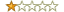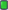#63
 contextraxFriendJoin Date: Aug 2017 Posts: 43 Rept. Given: 0 Rept. Rcvd 17 Times in 7 Posts Thanks Given: 4 Thanks Rcvd at 71 Times in 18 PostsQuote:
 Originally Posted by cjackThank's for sharing ECC Source contextrax! Excuse me for my ignorance but, how to calculate the 8 ecc_curve_array[] parameters of an armadillo protected application?
The first four of them does not need to be changed for a amradillo protected target.
They are the hamming weight, A in the koblitz curve equation (y^2 + xy = x^3 + Ax^2 + B), point order and cofactor.
The last four is the x,y coordinate for the base point and public point in polynomial base.
Armadillo uses a 32 bit's seed for the base point so you will have to use arma source code to calc these values. They are however i the Type 2 Optimal Normal Base and you will have to do base conversion of them to go to x^113+x^9+1
But for testing speed or making a linux or GPU version then this is not necessary.

The test curve that I use have A in the equation set to "0". This result in a different curve used for testing only. The order of this curve is 0x2000000000000000480DCA1242B1C and contains the factors:
Prime factor : 2 - (2 bits)
Prime factor : 2 - (2 bits)
Prime factor : 18B9 - (13 bits)
Prime factor : 1A89A0F - (25 bits)
Prime factor : 31F1F2A998BD31AF391 - (74 bits)
(Found by schoof2 from miracl library. "schoof2.exe 0 1 113 9")

This makes is pretty good for testing (coz of the low order) and the test curve base point has a order of 0x31F1F2A998BD31AF391. (74 bit)
You will also notice that the equivalence classes is maintained in all these subgroup (They all contain 113*2 as factors of p-1) which of course is typical for a koblitz curve.

For base conversion you could make a matrix B=[1, b, b^2....,b^(m-1)] (m=113 for this base)
Multiplying b^m*B^-1 should give you a polynomial base and to go from this base to x^113+x^9+1 you can use one of the 113 roots.
For root finding you can use magma or sage or some other algo if you want to make one yourself.

Hope this helps.

Last edited by contextrax; 02-20-2018 at 03:17.
 The Following 4 Users Say Thank You to contextrax For This Useful Post: alekine322 (10-25-2018), Apuromafo (03-04-2019), cjack (02-25-2018), tonyweb (02-25-2018)